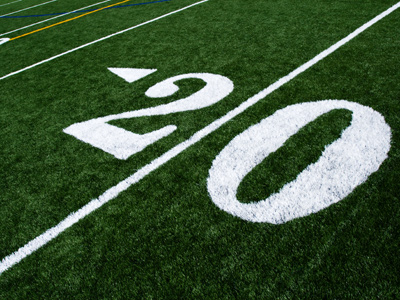Which numbers are less than 20?

# Algebra - Inequalities

This Math quiz is called 'Algebra - Inequalities' and it has been written by teachers to help you if you are studying the subject at middle school. Playing educational quizzes is a fabulous way to learn if you are in the 6th, 7th or 8th grade - aged 11 to 14.

It costs only \$12.50 per month to play this quiz and over 3,500 others that help you with your school work. You can subscribe on the page at Join Us

This algebra quiz deals with the relationships of one item to another; greater than, less than, the same as, etc., known as inequalities. First we look at the math symbols involved and then at some questions you will undoubtedly enjoy answering!

1.
< is the sign that means what?
Less than
Greater than
Less than or equal to
Greater than or equal to
This might help you remember which way the less than sign points: Less = Left (pointing)
2.
> is the sign that means what?
Less than
Greater than
Less than or equal to
Greater than or equal to
The small end of the sign always points to the smaller number
3.
≤ is the sign that means what?
Less than
Greater than
Less than or equal to
Greater than or equal to
Numbers that are < 6 are 5, 4, 3 etc; numbers that are ¡Ü 6 are 6, 5, 4, etc. Can you see the difference? If not then look again because it is important that you understand the difference between these signs
4.
≥ is the sign that means what?
Less than
Greater than
Less than or equal to
Greater than or equal to
5.
If x > 3, what is the smallest integer that x can be?
2
3
4
5
x must be greater than three and the next integer after three is four
6.
If x ≥ 19, which of the following numbers is not possible for the value of x?
18
19
20
21
7.
If 4x + 3 < 15, which of the following expressions are incorrect?
4x < 15 - 3
4x < 12
x < 3
x > 3
When a number crosses over the < or > signs then it changes from positive to negative or vise versa
8.
If 4x - 7 < 17, which of the following expressions are incorrect?
4x < 17 + 7
4x < 24
x > 6
x < 6
Values for x are less than 6
9.
If 16x - 5 > 59, which of the following is correct?
16x > 64
16x > 59
16x < 59
16x < 64
It is extremely easy to mix-up < and >, so be careful!
10.
If 9x + 40 > 140 which of these is correct?
9x - 40 > 140
9x > 100
9x < 100
x > 20
Author:  Frank Evans